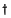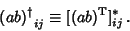The adjoint matrix, sometimes also called the Adjugate Matrix, is defined by(1)

where the Adjoint Operator is denotedanddenotes the Transpose. If a Matrix is Self-Adjoint, it is said to be Hermitian. The adjoint matrix of a Matrix product is given by(2)

Using the property of transpose products that(3)

it follows that(4)